## 6.3.4. Accumulate Functions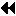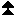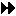Up: Communication Calls Next: Synchronization Calls Previous: Examples

It is often useful in a put operation to combine the data moved to the target process with the data that resides at that process, rather then replacing the data there. This will allow, for example, the accumulation of a sum by having all involved processes add their contribution to the sum variable in the memory of one process.

MPI_ACCUMULATE(origin_addr, origin_count, origin_datatype, target_rank, target_disp, target_count, target_datatype, op, win)
[ IN origin_count] number of entries in buffer (nonnegative integer)
[ IN origin_datatype] datatype of each buffer entry (handle)
[ IN target_rank] rank of target (nonnegative integer)
[ IN target_disp] displacement from start of window to beginning of target buffer (nonnegative integer)
[ IN target_count] number of entries in target buffer (nonnegative integer)
[ IN target_datatype] datatype of each entry in target buffer (handle)
[ IN op] reduce operation (handle)
[ IN win] window object (handle)

int MPI_Accumulate(void *origin_addr, int origin_count, MPI_Datatype origin_datatype, int target_rank, MPI_Aint target_disp, int target_count, MPI_Datatype target_datatype, MPI_Op op, MPI_Win win)

MPI_ACCUMULATE(ORIGIN_ADDR, ORIGIN_COUNT, ORIGIN_DATATYPE, TARGET_RANK, TARGET_DISP, TARGET_COUNT, TARGET_DATATYPE, OP, WIN, IERROR)
INTEGER ORIGIN_COUNT, ORIGIN_DATATYPE,TARGET_RANK, TARGET_COUNT, TARGET_DATATYPE, OP, WIN, IERROR

void MPI::Win::Accumulate(const void* origin_addr, int origin_count, const MPI::Datatype& origin_datatype, int target_rank, MPI::Aint target_disp, int target_count, const MPI::Datatype& target_datatype, const MPI::Op& op) const

Accumulate the contents of the origin buffer (as defined by origin_addr, origin_count and origin_datatype) to the buffer specified by arguments target_count and target_datatype, at offset target_disp, in the target window specified by target_rank and win, using the operation op. This is like MPI_PUT except that data is combined into the target area instead of overwriting it.

Any of the predefined operations for MPI_REDUCE can be used. User-defined functions cannot be used. For example, if op is MPI_SUM, each element of the origin buffer is added to the corresponding element in the target, replacing the former value in the target.

Each datatype argument must be a predefined datatype or a derived datatype, where all basic components are of the same predefined datatype. Both datatype arguments must be constructed from the same predefined datatype. The operation op applies to elements of that predefined type. target_datatype must not specify overlapping entries, and the target buffer must fit in the target window.

A new predefined operation, MPI_REPLACE, is defined. It corresponds to the associative function f(a,b) = b; i.e., the current value in the target memory is replaced by the value supplied by the origin.

MPI_PUT is a special case of MPI_ACCUMULATE, with the operation MPI_REPLACE. Note, however, that MPI_PUT and MPI_ACCUMULATE have different constraints on concurrent updates. ( End of advice to users.)

Example We want to compute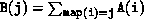. The arrays A, B and map are distributed in the same manner. We write the simple version.

```SUBROUTINE SUM(A, B, map, m, comm, p)
USE MPI
INTEGER m, map(m), comm, p, sizeofreal, win, ierr
REAL A(m), B(m)

CALL MPI_TYPE_EXTENT(MPI_REAL, sizeofreal, ierr)
CALL MPI_WIN_CREATE(B, m*sizeofreal, sizeofreal, MPI_INFO_NULL,  &
comm, win, ierr)

CALL MPI_WIN_FENCE(0, win, ierr)
DO i=1,m
j = map(i)/p
k = MOD(map(i),p)
CALL MPI_ACCUMULATE(A(i), 1, MPI_REAL, j, k, 1, MPI_REAL,   &
MPI_SUM, win, ierr)
END DO
CALL MPI_WIN_FENCE(0, win, ierr)

CALL MPI_WIN_FREE(win, ierr)
RETURN
END
```
This code is identical to the code in Example Examples , except that a call to get has been replaced by a call to accumulate. (Note that, if map is one-to-one, then the code computes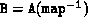, which is the reverse assignment to the one computed in that previous example.) In a similar manner, we can replace in Example Examples , the call to get by a call to accumulate, thus performing the computation with only one communication between any two processes.Up: Communication Calls Next: Synchronization Calls Previous: Examples